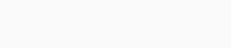## Physics

Question 1.
Monochromatic light of wavelength 589 nm is incident from air on a water surface. What are the wavelength, frequency and speed of
(a) reflected, and
(b) refracted light? Refractive index of water is 1.33.
Solution:
(a) In the process of reflection wavelength, frequency and speed of incident light remain unchanged. So, speed of reflected light = speed of incident light(b) In the process of refraction wavelength and speed changes but the frequency remain the same. Speed of light in waterQuestion 2.
What is the shape of the wave front in each of the following cases:
(a) Light diverging from a point source.
(b) Light emerging out of a convex lens when a point source is placed at its focus.
(c) The portion of the wave front of light from a distant star intercepted by the Earth.
Solution:
(a) Spherical wave front : All particles vibrating in same phase will lie on a sphere.
(b) Plane wave front: Light will be a parallel beam after passing through the convex lens.
(c) Plane wave front : Light rays from a distant star are nearly parallel as a small portion of a huge spherical wave front is nearly plane.

Question 3.
(a) The refractive index of glass is 1.5. What is the speed of light in glass? (Speed of light in vacuum is 3.0 × 108 m s-1)
(b) Is the speed of light in glass independent of the colour of light? If not, which of the two colours, red and violet, travels slower in a glass prism?
Solution:
(a) Speed of light in glass,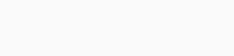(b) Yes, speed of light in glass depends upon the colour of light (i.e., λ). Thus, speed of light is different for red and violet colours. As µv > µR so, λv < λR hence, υv < υR Speed of red colour is more than violet colour light in glass.

Question 4.
In a Young’s double-slit experiment, the slits are separated by 0.28 mm and the screen is placed 1.4 m away. The distance between the central bright fringe and the fourth bright fringe is measured to be 1.2 cm. Determine the wavelength of light used in the experiment.
Solution:
Here d = 0.28 mm, D = 1.4 m Distance of fourth bright fringe from centerQuestion 5.
In Young’s double-slit experiment using monochromatic light of wavelength X, the intensity of light at a point on the screen where path difference is X, is K units. What is the intensity of light at a point where path difference is λ/3?
Solution:
In Young’s double-slit experiment net intensity of light at a point on screen isQuestion 6.
A beam of light consisting of two wavelengths, 650 nm and 520 nm is used to obtain interference fringes in a Young’s double-slit experiment.
(a) Find the distance of the third bright fringe on the screen from the central maximum for wavelength 650 nm.
(b) What is the least distance from the central maximum where the bright fringes due to both the wavelengths coincide?
Solution:(a) Distance of third bright fringe from the central maximum for the wavelength 650 nm.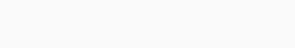(b) Let at linear distance ‘y’ from center of screen the bright fringes due to both wavelength coincides. Let n1 number of bright fringe with wavelength λ1 coincides with n2 number of bright fringe with wavelength λ2. We can writeAlso at first position of coincide, the nth bright fringe of one will coincide with (n + l) th bright fringe of other.So, the fourth bright fringe of wavelength 520 nm coincides with 5th bright fringe of wavelength 650 nm.

Question 7.
In a double-slit experiment the angular width of a fringe is found to be 0.2° on a screen placed 1 m away. The wavelength of light used is 600 nm. What will be the angular width of the fringe if the entire experimental apparatus is immersed in water? Take refractive index of water to be 4/3.
Solution:

Question 8.

What is the Brewster angle for air to glass transition? (p for glass is 1.5)
Solution: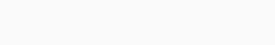Question 9.
Light of wavelength 5000 A falls on a plane reflecting surface. What are the wavelength and frequency of the reflected light? For what angle of incidence is the reflected ray normal to the incident ray?
Solution:
In the reflected light the wavelength and frequency remain the same as that of incident light. Wavelength of reflected light = 5000 A, frequency of reflected lightFor an angle of incidence 45° the reflected ray is normal to incident ray.

Question 10.
Estimate the distance for which ray optics is good approximation for an aperture of 4 mm and wavelength 400 nm.
Solution:
Fresnel distance required for a sufficient spreading of central bright fringe, so that diffraction is appreciable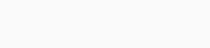So, for distance less than 40 m between slit and screen, ray optics is a good approximation as within this distance, the spreading is negligible.

Question 11.
The 6563 A Ha line emitted by hydrogen in a star is found to be red shifted by 15 A. Estimate the speed with which the star is receding from the earth.
Solution: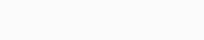Since the star is receding away, hence its velocity v is negative (i.e. if AA is positive, v is negative)Here, negative sign shows recession of star.

Question 12.
Explain how corpuscular theory predicts that the speed of light in a medium, say, water, is greater than the speed of light in vacuum. Is the prediction confirmed by experimental determination of the speed of light in water? If not, which alternative picture of light is consistent with experiment?
Solution:
In Newton’s corpuscular (particle) picture of refraction, particles of light incident from a rarer to a denser medium experience a force of attraction normal to the surface. This results in an increase in the normal component of velocity but the component along the surface remains unchanged.Considering a ray of light going from a rarer medium (air) to a denser medium (water). Let c = speed of light in vacuum (or air), v = speed of light in water, i = angle of incidence, and r = angle of refraction Then according to Newton’s corpuscular theory,

Component of velocity c along surface of separation = Component of velocity v along the surface of separationSo, according to Newton’s corpuscular theory the speed of light in medium is larger than speed of light in air. υ > c but in fact the experimental observation shows that speed of light is smaller in denser medium as compared to rare medium υ < c.

Question 13.
You have learnt in the text how Huygens principle leads to the laws of reflection and refraction. Use the same principle to deduce directly that a point object placed in front of a plane mirror produces a virtual image whose distance from the mirror is equal to the distance of the object from the mirror.
Solution:In figure, P is a point object placed at a distance . r from a plane mirror M1M2. With P as centre and PO = r as radius, draw a spherical arc; AB. This is the spherical wave front from the object, incident on M1M2 If mirrors were not present, the position of wave front AB would be A’B’ where PP’ = 2r. In the presence of the mirror, wave front A B would appear as A”PB”, according to Huygen’s construction. As it is clear from the figure A’B’ and A”B” are two spherical arcs located symmetrically on either side of M1M2. , Therefore, A’P’B’ can be treated as reflected image of A”PB”. From simple geometry, we find OP = OP’, which was to be proved.

Question 14.
Let us list some of the factors, which could possibly influence the speed of wave propagation:
(i) nature of the source.
(ii) direction of propagation.
(iii) motion of the sources and/or observer.
(iv) wavelength.
(v) intensity of the wave. On which of these factors, if any, does
(a) the speed of light in vacuum,
(b) the speed of light in a medium (say glass or water) depend?
Solution:
(a) Speed of light in vacuum is independent of all the factors listed above. It is also independent of relative motion between source and observer.
(b) Dependence of speed of light in a medium.
(i) The speed of light in a medium does not depend on the nature of the source. Although speed is determined by the properties of the medium of propagation.
(ii) The speed of light in a medium is independent of the direction of propagation for an isotropic media.
(iii) The speed of light is independent of the motion of the source relative to the medium but it depends upon the motion of the observer relative to the medium.
(iv) The speed of light in a medium depends on wavelength of light i.e., v ∝ λ..
(v) The speed of light in a medium is independent of intensity.

Question 15.
For sound waves, the Doppler formula for frequency shift differs slightly between the two situations :
(i) source at rest; observer moving, and
(ii) source moving; observer at rest.
The exact Doppler formulas for the case of light waves in vacuum are, however, strictly identical for these situations. Explain why this should be so. Would you expect the formulas to be strictly identical for the two situations in case of light travelling in a medium?
Solution:
Sound waves require a medium for propagation. The Doppler formula for frequency shift differs slightly in two situations
(i) Source at rest, observer moving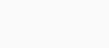(ii) Observer at rest, source moving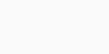The two formulas are different because motion of the observer relative to the medium is different in the two situations for light waves in vacuum. No such relative relation of observer and medium exist. Hence only the relative motion between the source and the observer counts and the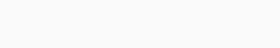Question 16.
In double-slit experiment using light of wavelength 600 nm, the angular width of a fringe formed on a distant screen is 0.1°. What is the spacing between the two slits?
Solution:

Question 17.

(a) In a single slit diffraction experiment, the width of the slit is made double the original width. How does this affect the size and intensity of the central diffraction band?
(b) In what way is diffraction from each slit related to the interference pattern in a double-slit experiment?
(c) When a tiny circular obstacle is placed in the path of light from a distant source, a bright spot is seen at the centre of the shadow of the obstacle. Explain why?
(d) Two students are separated by a 7 m partition wall in a room 10 m high. If both light and sound waves can bend around obstacles, how is it that the students are unable to see each other even though they can converse easily.
(e) Ray optics is based on the assumption that light travels in a straight line. Diffraction effects (observed when light propagates through small apertures/slits or around small obstacles) disprove this assumption. Yet the ray optics assumption is so commonly used in understanding location and several other properties of images in optical instruments. What is the justification?
Solution:
(a) Linear width of central maximum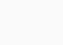On doubling the slit width ‘d’, the size of central diffraction band is halved.
Because the width of central maximum is halved. Its area become 1/4 times and hence the intensity become 4 times the initial intensity.
(b) In double slit experiment, an interference pattern is observed by waves from two slits but as each slit provide a diffraction pattern of its own, thus the intensity of interference pattern in Young’s double slit experiment is modified by diffraction pattern of each slit.
(c) Waves from the distant source are diffracted by the edge of the circular obstacle and these waves superimpose constructively at the centre of obstacle’s shadow producing a bright spot.
(d) We know for diffraction to take place, size of the obstacle/aperture should be of the order of wavelength. Wavelength of sound waves is of the order of few meters that is why sound waves can bend through the aperture in partition wall but wavelength of light waves is of the order of micrometer, hence light waves can not bend through same big aperture. That is why the two students can hear each other but cannot see each other.(e) In optical instruments, the sizes of apertures are much larger as compared to wavelength of light. So the diffraction effects are negligibly small. Hence, the assumption that light travels in straight lines is used in the optical instruments.

Question 18.
Two towers on top of two hills are 40 km apart. The line joining them passes 50 m above a hill halfway between the towers. What is the longest wavelength of radio waves, which can be sent between the towers without appreciable diffraction effects?
Solution:

For diffraction of radio waves not to occur the distance of middle hill should be less than fresnel distance for a slit width ‘a’ of 50 m.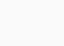Distance between one of the towers and the hill halfway in between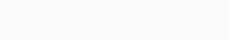Longest wavelength of radio wave which can be sent without appreciable diffraction effect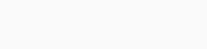Thus wavelength of radio waves longer than 12.5 cm will bend due to the hill in the middle of towers.

Question 19.
A parallel beam of light of wavelength 500 nm falls on a narrow slit and the resulting diffraction pattern is observed on a screen 1 m away. It is observed that the first minimum is at a distance of 2.5 mm from the centre of the screen. Find the width of the slit.
Solution:

First minimum is observed at a distance 2.5 mm from centre of the screen.

Question 20.